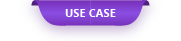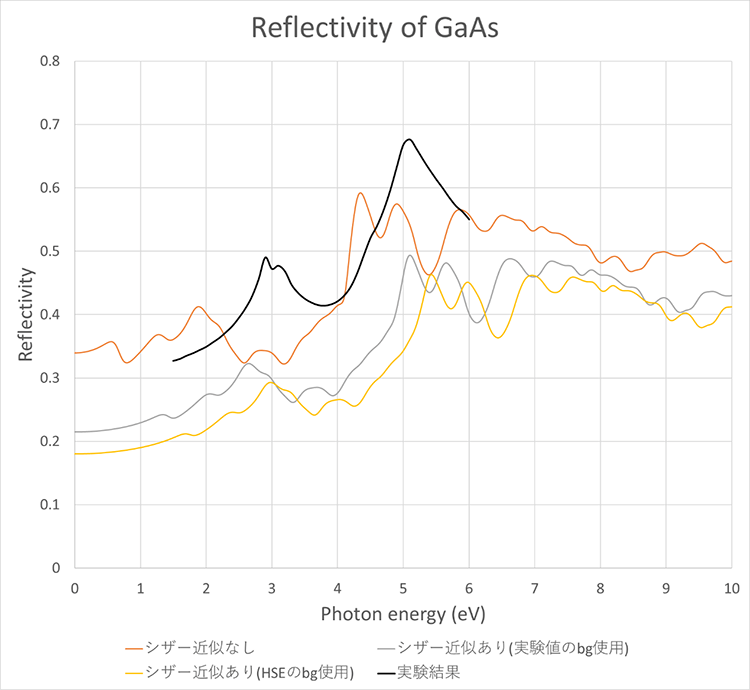Since III-V semiconductors are used as materials for light emitting devices such as diodes, it is useful to predict their optical characteristics from first-principles calculations.

# Analysis of optical properties of III-V semiconductors

Since III-V semiconductors are used as materials for light emitting devices such as diodes, it is useful to predict their optical characteristics from first-principles calculations. Here, we explain how to calculate the dielectric function of Si and GaAs, which is a group III-V semiconductor, using first-principles calculation. It is also possible to evaluate optical properties such as absorption spectrum, permittivity, and reflectance from the dielectric function .

#### 1. Computational model creation

You can easily obtain the required crystal structure by searching for the substance names Si and GaAs on the Import Materials screen (Fig. 1 and Fig. 2).

#### 2. Execution of electronic dielectric function calculation

The electronic dielectric function is calculated using epsilon.x, which is the dielectric function calculation function of Quantum Espresso. Table 1 shows the calculation conditions for calculating the dielectric function in epsilon.x.

###### Table 1 Dielectric function calculation conditions
itemdetail
PseudopotentialSi_ONCV_PBE_sr.upf
Ga_ONCV_PBE_sr.upf
As_ONCV_PBE_sr.upf
Cutoff wave function55 Ry
Cutoff electron density220 Ry
k pointscf: 4 × 4 × 4
nscf: 12 × 12 × 12
Convergence threshold10 -10
Number of bands20

##### 2.1 Absorption spectrum

Figures 3 and 4 show the calculation results of the imaginary part of the dielectric function. The imaginary part of the dielectric function corresponds to the absorption spectrum. By performing scissor approximation, it can be confirmed that the absorption position of the spectrum approaches the experimental result.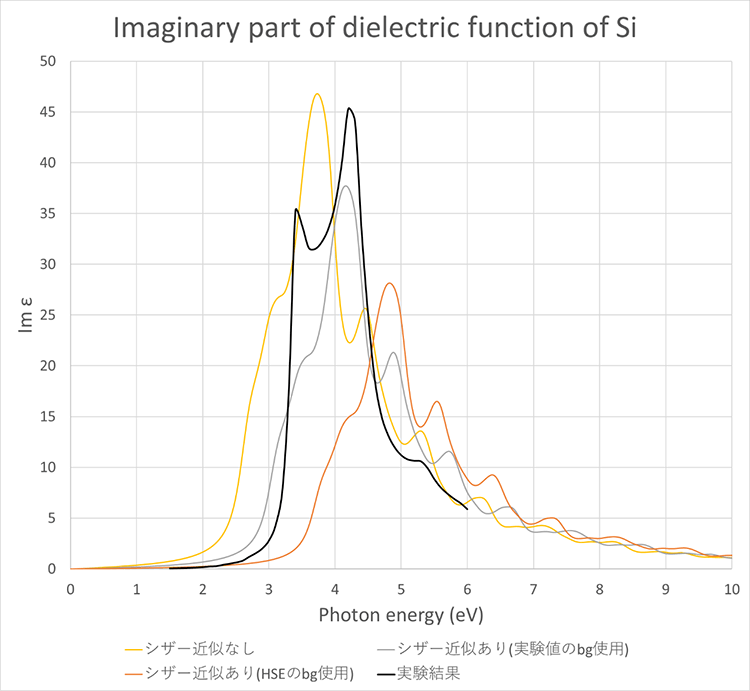Fig. 3 Comparison of the calculation result of the absorption spectrum (imaginary part of the dielectric function) of Si and the experimental result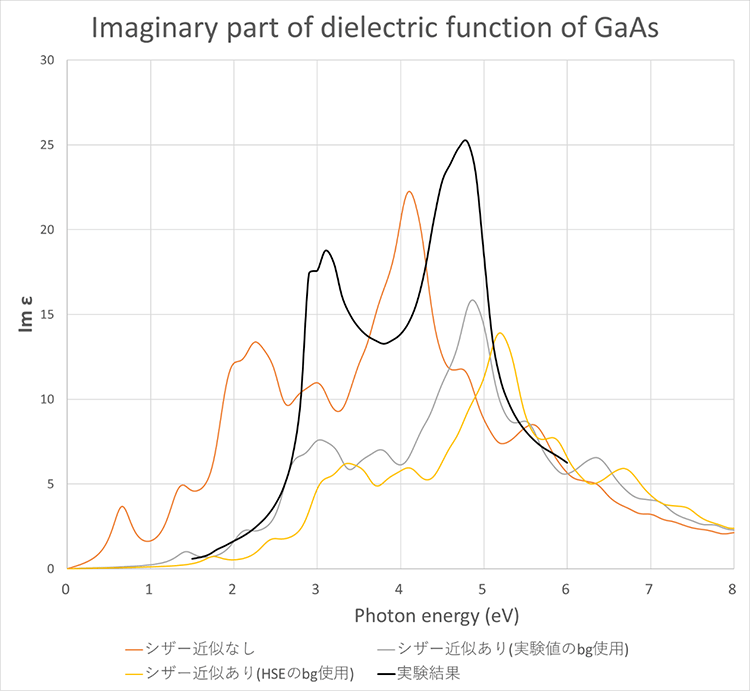Fig. 4 Comparison of the calculation result of the absorption spectrum (imaginary part of the dielectric function) of GaAs and the experimental result 

##### 2.2 Permittivity

In addition, Fig. 5 and Fig. 6 show the calculation results of the real part of the dielectric function. The permittivity can be evaluated from the value of the dielectric function when Photon energy is 0. Table 4 shows the calculation results of the permittivity. The shape of the dielectric function (peak position, etc.) is closer to the experimental result by performing scissor approximation. On the other hand, the value of the dielectric function was improved by scissor approximation in Si, but not in GaAs.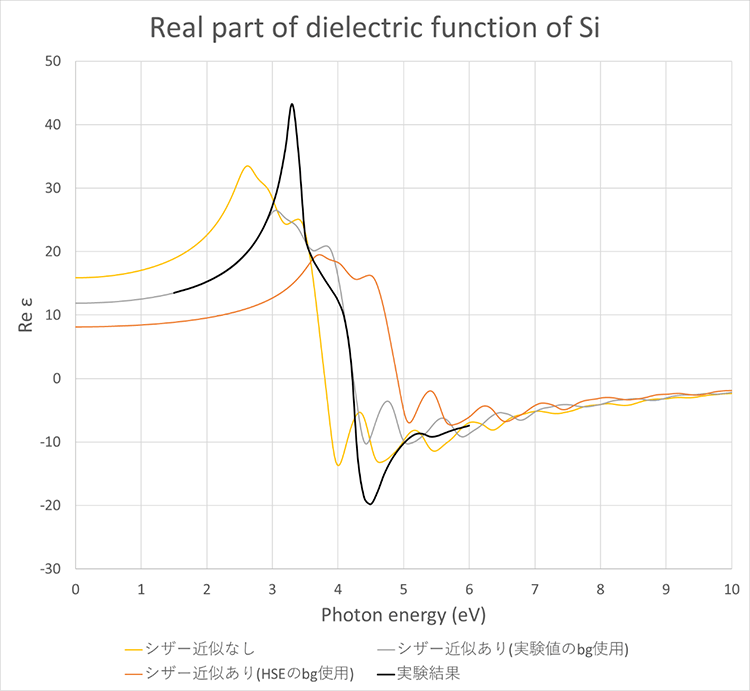Fig. 5 Comparison of the calculation result of the real part of the dielectric function of Si and the experimental result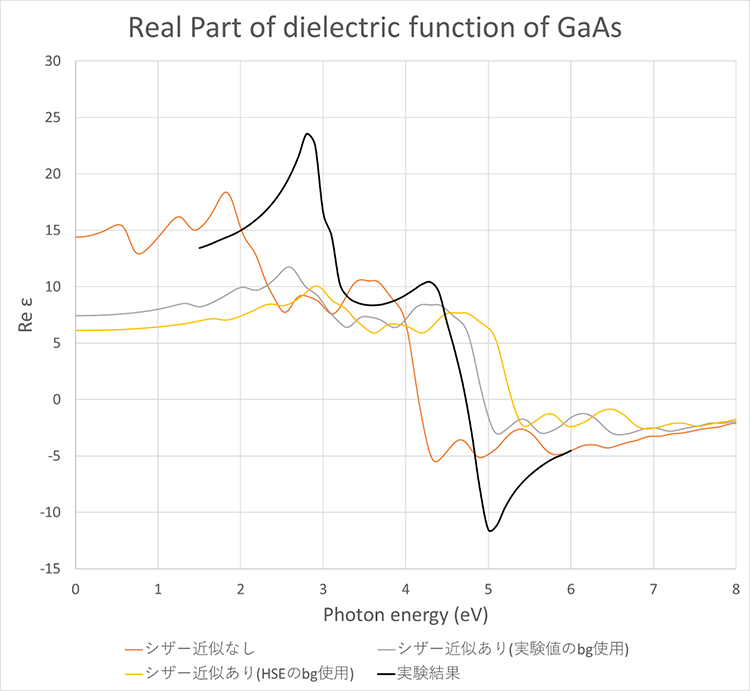Fig. 6 Comparison of the calculation result of the real part of the dielectric function of GaAs and the experimental result 

##### 2.3 Reflectance

Next, Fig. 7 and Fig. 8 show the calculation results of the reflectance. By performing scissor approximation, it can be confirmed that the shape of the reflectance (peak position, etc.) approaches the experimental result. In the case of Si, the reflectance value was close to the experimental result by performing scissor approximation.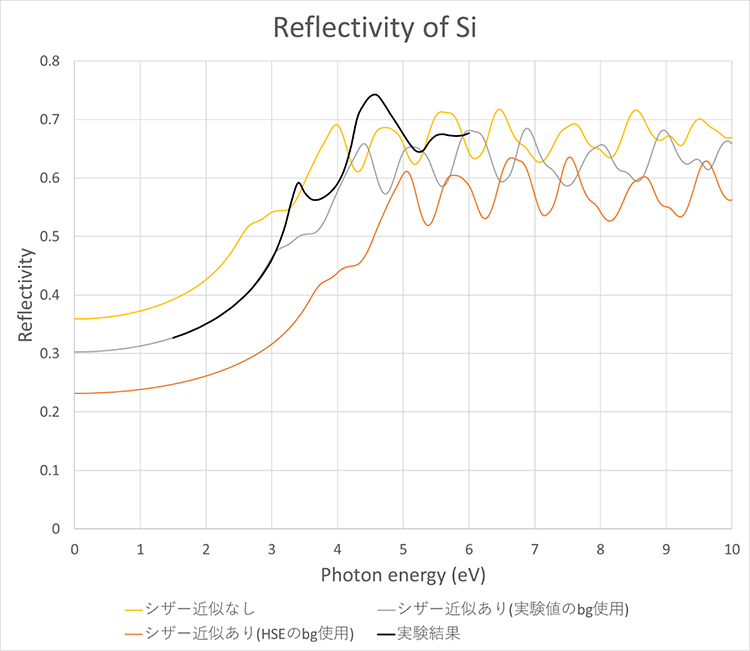Fig. 7 Comparison of the calculation result of the reflectance of Si and the experimental result 

#### 3. About the workflow of electronic dielectric function calculation

Table 5 shows the workflow (Figure 9) and detailed information for calculating the electronic dielectric function in epsilon.x of Quantum Espresso.

###### Table 5 Workflow information for permittivity calculation

In addition, the energy range of the graph output can be set by inputting the lower and upper limits of energy during the mk_graph flow (Fig. 10).

Fig. 10 Graph drawing execution flow Energy range input field in mk_graph

Emin corresponds to the energy lower limit value and Emax corresponds to the energy upper limit value (eV unit)

For the workflow of the electronic dielectric function, obtain "electronic_dielectric_function (QE6.3)" from Bank and use it.

#### 4. Calculation time and cost

Finally, the calculation time and cost required to carry out this calculation are shown in Table 6.

Table 6 Calculation time and cost of electronic dielectric function calculation (using saving node)

#### References

Original Source from: https://ctc-mi-solution.com/iii-v半導体の光学特性の解析/